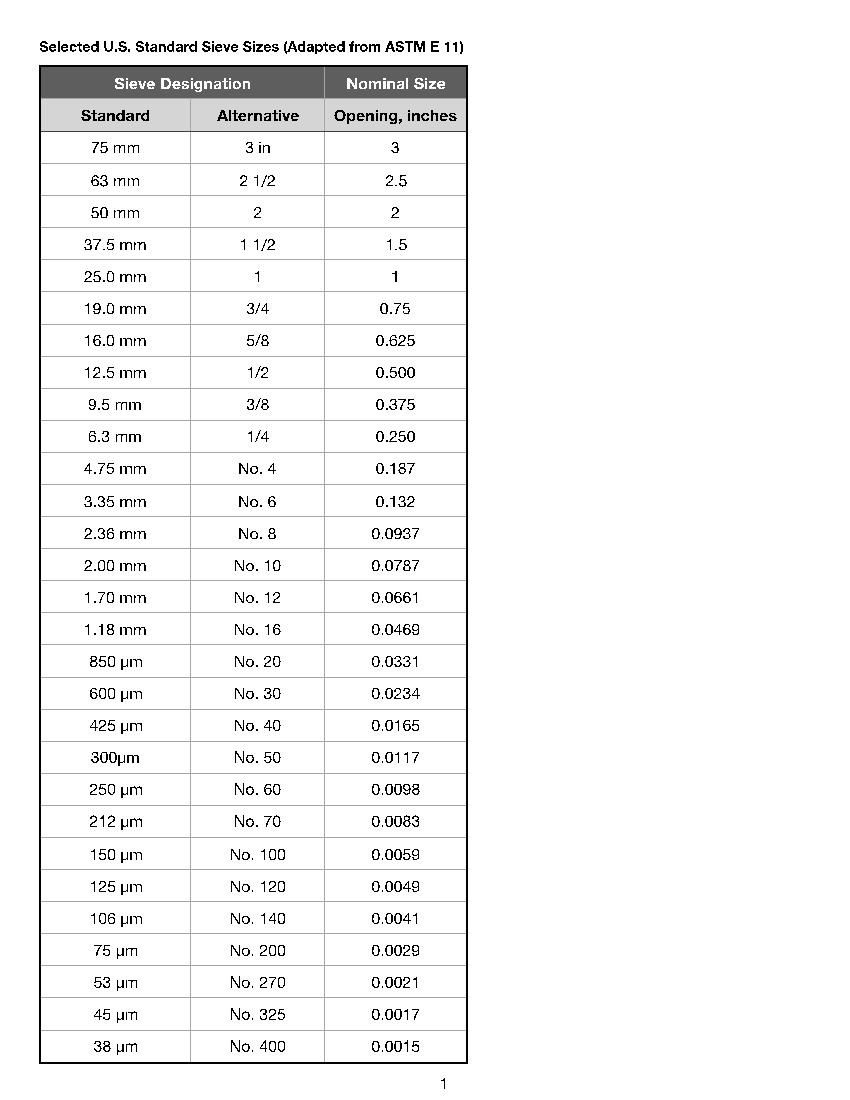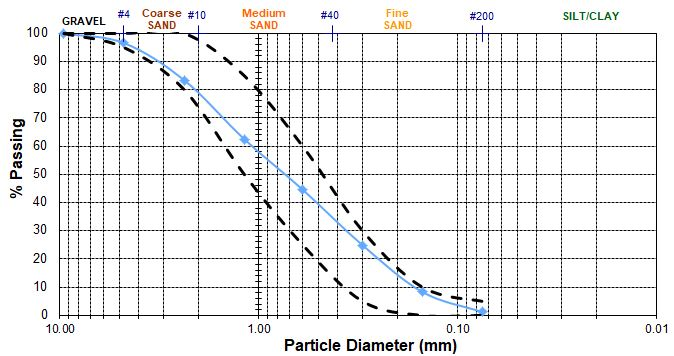# Gradations, D-Values & Uniformity Coefficients: What does it all mean?

Back to Blog Home

Gradations, D-Values & Uniformity Coefficients: What does it all mean?

Gradations, D-values and uniformity coefficients are all components of particle size distribution and analysis of aggregate materials. Particle size analysis is extremely important in order to determine the cost, quality, and performance of a particular aggregate for the project at hand. For example, silica sand used for water filtration requires clean, kiln dried, uniformly shaped grains with a narrow range of particle sizes whereas sand used in concrete applications requires washed, non-reactive, and well-graded material that meets CSA Standards. Generally, the more processed the aggregate, the higher the quality and cost of the product. This is because of labour, equipment, and quality control costs, as well as the abundance of material loss during processing. Practises that add to material costs and value to the customer include the washing, drying, screening, storing, and packaging of materials. For more information on typical step-by-step processing of aggregates, see the infographic below:Particle size analysis is generally performed using mechanical sieving, which divides and weighs a sample of aggregate into fractions, each consisting of particles of the same size (between specific limits determined by the set of screen sizes used – see figure 1) represented as cumulative percent retained on the sieve and cumulative percent passing through the sieve (see figure 2), and generates data used to present particle size information in the form of an S-curve (see figure 3).

Figure 1: Selected standard sieve sizes. Common aggregates have a defined set of sieves that determine the gradation (size distribution) and quality of the desired product.Figure 2: Example Sieve analysis chartFigure 3: Example S-Curve Diagram (gradation curve)The S-curve (often referred to as a gradation curve diagram) is logarithmic graphical representation of cumulative percent passing of grains on the y-axis, and the sieve size opening on the x-axis.

Once you have your S-curve plotted, you can calculate three important qualities about your sample:

1. Effective Size – the average diameter of particles in a granular sample. D-Values are used to describe the effective size, based on the amount of aggregate which passes through a defined sieve:
1. D60 Sieve opening size (mm) through which 60% of aggregate passes
2. D10 Sieve opening size (mm) through which 10% of aggregate passes
2. Uniformity Coefficient (AKA Coefficient of Uniformity or Cu) – the ratio of D60 to D10, which is the slope of the grainsize distribution curve of your sample.

Cu= D60/D10

1. Cu > 6 indicates a densely graded (i.e. well-graded) material with a considerable range of particle size (used for max stability).
2. Cu < 4 indicates a uniformly graded (i.e. open-graded) material having a narrow range of particle size (used for max permeability).
1. Fineness Modulus – an index describing the fineness or coarseness of an aggregate. It is calculated by adding up the cumulative percent of retained aggregate on each sieve (from a samples sieve analysis chart – ex. figure 2, above) and dividing it by 100.

This is exactly what you need to know about gradations, D-values, and uniformity coefficients and how it impacts costs and performance.